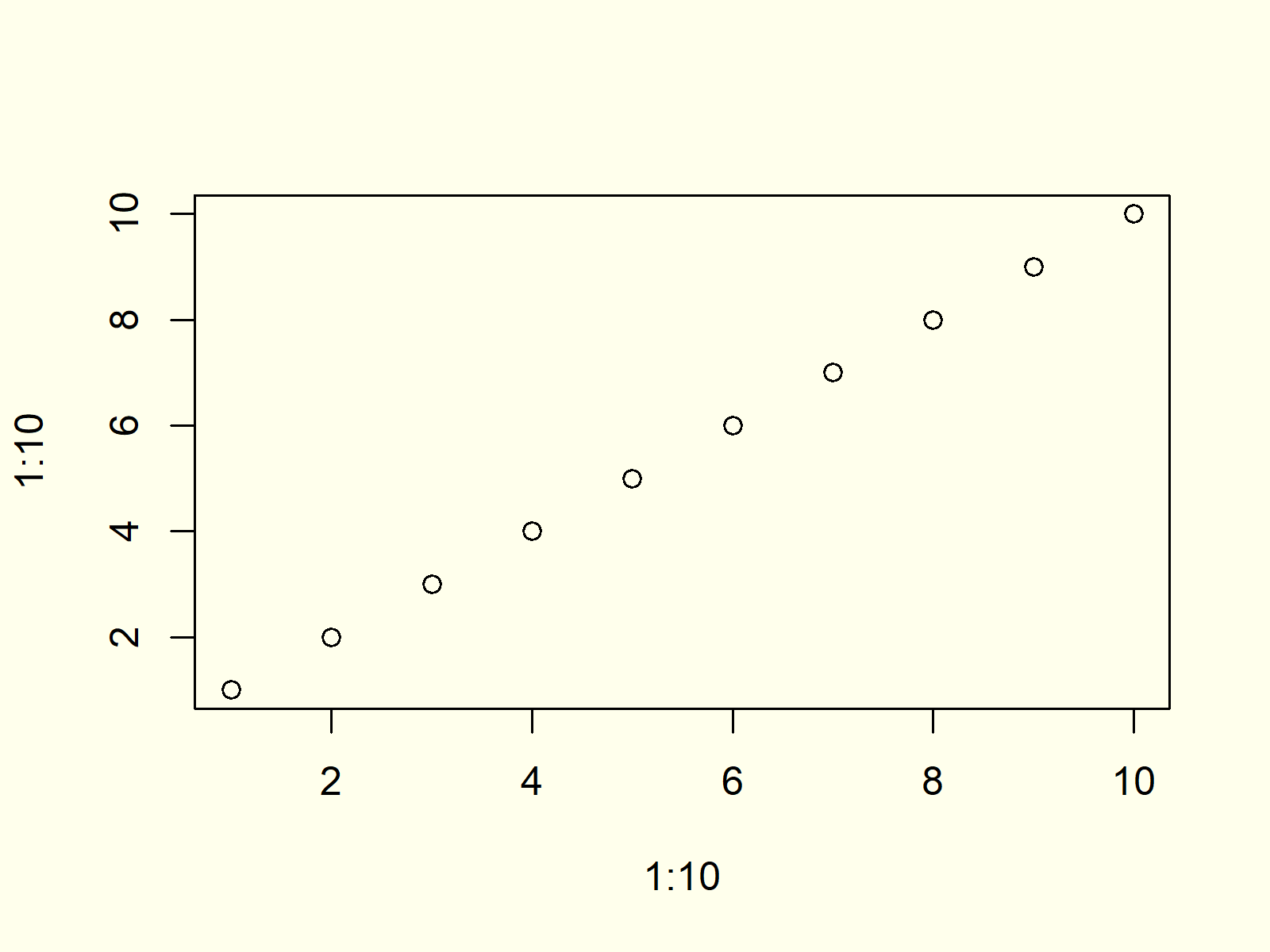# Control the Size of the Points in a Scatterplot in R (Example)

In this article, I’ll show how to increase or decrease the point size in a scatterplot in the R programming language.

The tutorial will consist of one example. To be more precise, the tutorial will contain the following content blocks:

Let’s just jump right in:

## Default Scatterplot

In R, we can draw a default scatterplot (or xy-plot) with the plot() function as follows:

`plot(1:10, 1:10)              # Create default scatterplot`Figure 1: Default Scatterplot in R.

Figure 1 shows how a default scatterplot in R looks like. As you can see, the points are relatively small.

## Example: Increase Point Size of Plot

If we want to increase the size of the points of our plot, we can use the cex argument of the plot function:

`plot(1:10, 1:10, cex = 3)     # Increase size of points`Figure 2: Scatterplot with Increased Size of Points.

Compare the points shown in Figure 2 with the points shown in Figure 1. As you can see, the point size was increased.

Note that we could specify any numeric value for the cex argument. Smaller values indicate a smaller point size; larger values indicate a larger point size.

## Video, Further Resources & Summary

Would you like to learn more about plotting data in R? Then you might want to have a look at the following video of my YouTube channel. I explain the R programming syntax of this tutorial in the video.

In addition, you might have a look at the other articles of www.statisticsglobe.com.

At this point of the post you should know how to control and modify the size and thickness of the dots in a xy-plot in R. Please let me know in the comments, if you have any additional questions and/or comments.

Subscribe to the Statistics Globe Newsletter